# Maharashtra Board 12th Maths Solutions Chapter 4 Pair of Straight Lines Ex 4.2

Balbharti 12th Maharashtra State Board Maths Solutions Book Pdf Chapter 4 Pair of Straight Lines Ex 4.2 Questions and Answers.

## Maharashtra State Board 12th Maths Solutions Chapter 4 Pair of Straight Lines Ex 4.2

Question 1.
Show that lines represented by 3x2 – 4xy – 3y2 = 0 are perpendicular to each other.
Solution:
Comparing the equation 3x2 – 4 xy – 3y2 = 0 with ax2 + 2hxy + by2 = 0, we get, a = 3, 2h = -4, b = -3 Since a + b = 3 + (-3) = 0, the lines represented by 3x2 – 4xy – 3y2 = 0 are perpendicular to each other.

Question 2.
Show that lines represented by x2 + 6xy + gy2= 0 are coincident.
The question is modified.
Show that lines represented by x2 + 6xy + 9y2= 0 are coincident.
Solution:
Comparing the equation x2 + 6xy + 9y2 = 0 with ax2 + 2hxy + by2 = 0, we get,
a = 1, 2h = 6, i.e. h = 3 and b = 9
Since h2 – ab = (3)2 – 1(9)
= 9 – 9 = 0, .
the lines represented by x2 + 6xy + 9y2 = 0 are coincident.Question 3.
Find the value of k if lines represented by kx2 + 4xy – 4y2 = 0 are perpendicular to each other.
Solution:
Comparing the equation kx2 + 4xy – 4y2 = 0 with ax2 + 2hxy + by2 = 0, we get,
a = k, 2h = 4, b = -4
Since lines represented by kx2 + 4xy – 4y2 = 0 are perpendicular to each other,
a + b = 0
∴ k – 4 = 0 ∴ k = 4.

Question 4.
Find the measure of the acute angle between the lines represented by:
(i) 3x2 – 4$$\sqrt {3}$$xy + 3y2 = 0
Solution:
Comparing the equation 3x2 – 4$$\sqrt {3}$$xy + 3y2 = 0 with
ax2 + 2hxy + by2 = 0, we get,
a = 3, 2h = -4$$\sqrt {3}$$, i.e. h = -24$$\sqrt {3}$$ and b = 3
Let θ be the acute angle between the lines.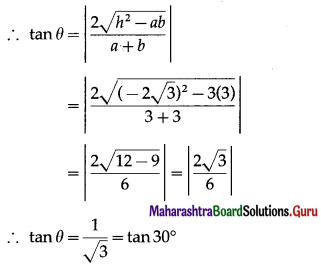∴ θ = 30°.(ii) 4x2 + 5xy + y2 = 0
Solution:
Comparing the equation 4x2 + 5xy + y2 = 0 with ax2 + 2hxy + by2 = 0, we get,
a = 4, 2h = 5, i.e. h = $$\frac{5}{2}$$ and b = 1.
Let θ be the acute angle between the lines.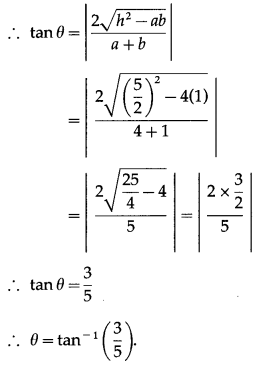(iii) 2x2 + 7xy + 3y2 = 0
Solution:
Comparing the equation
2x2 + 7xy + 3y2 = 0 with
ax2 + 2hxy + by2 = 0, we get,
a = 2, 2h = 7 i.e. h = $$\frac{7}{2}$$ and b = 3
Let θ be the acute angle between the lines.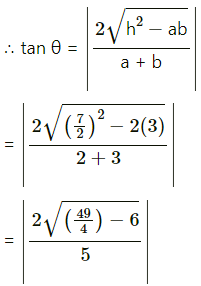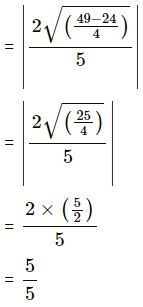tanθ = 1
∴ θ = tan 1 = 45°
∴ θ = 45°(iv) (a2 – 3b2)x2 + 8abxy + (b2 – 3a2)y2 = 0
Solution:
Comparing the equation
(a2 – 3b2)x2 + 8abxy + (b2 – 3a2)y2 = 0, with
Ax2 + 2Hxy + By2 = 0, we have,
A = a2 – 3b2, H = 4ab, B = b2 – 3a2.
∴ H2 – AB = 16a2b2 – (a2 – 3b2)(b2 – 3a2)
= 16a2b2 + (a2 – 3b2)(3a2 – b2)
= 16a2b2 + 3a4 – 10a2b2 + 3b4
= 3a4 + 6a2b2 + 3b4
= 3(a4 + 2a2b2 + b4)
= 3 (a2 + b2)2
∴ $$\sqrt{H^{2}-A B}$$ = $$\sqrt {3}$$ (a2 + b2)
Also, A + B = (a2 – 3b2) + (b2 – 3a2)
= -2 (a2 + b2)
If θ is the acute angle between the lines, then
tan θ = $$\left|\frac{2 \sqrt{H^{2}-A B}}{A+B}\right|=\left|\frac{2 \sqrt{3}\left(a^{2}+b^{2}\right)}{-2\left(a^{2}+b^{2}\right)}\right|$$
= $$\sqrt {3}$$ = tan 60°
∴ θ = 60°

Question 5.
Find the combined equation of lines passing through the origin each of which making an angle of 30° with the line 3x + 2y – 11 = 0
Solution:
The slope of the line 3x + 2y – 11 = 0 is m1 = $$-\frac{3}{2}$$ .
Let m be the slope of one of the lines making an angle of 30° with the line 3x + 2y – 11 = 0.
The angle between the lines having slopes m and m1 is 30°.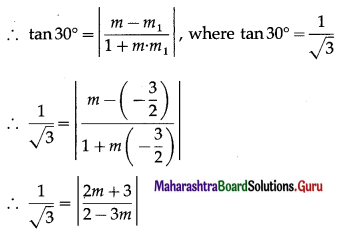On squaring both sides, we get,
$$\frac{1}{3}=\frac{(2 m+3)^{2}}{(2-3 m)^{2}}$$
∴ (2 – 3m)2 = 3 (2m + 3)2
∴ 4 – 12m + 9m2 = 3(4m2 + 12m + 9)
∴ 4 – 12m + 9m2 = 12m2 + 36m + 27
3m2 + 48m + 23 = 0
This is the auxiliary equation of the two lines and their joint equation is obtained by putting m = $$\frac{y}{x}$$.
∴ the combined equation of the two lines is
3$$\left(\frac{y}{x}\right)^{2}$$ + 48$$\left(\frac{y}{x}\right)$$ + 23 = 0
∴ $$\frac{3 y^{2}}{x^{2}}+\frac{48 y}{x}$$ + 23 = 0
∴ 3y2 + 48xy + 23x2 = 0
∴ 23x2 + 48xy + 3y2 = 0.Question 6.
If the angle between lines represented by ax2 + 2hxy + by2 = 0 is equal to the angle between lines represented by 2x2 – 5xy + 3y2 = 0 then show that 100(h2 – ab) = (a + b)2.
Solution:
The acute angle θ between the lines ax2 + 2hxy + by2 = 0 is given by
tan θ = $$\left|\frac{2 \sqrt{h^{2}-a b}}{a+b}\right|$$ ..(1)
Comparing the equation 2x2 – 5xy + 3y2 = 0 with ax2 + 2hxy + by2 = 0, we get,
a = 2, 2h= -5, i.e. h = $$-\frac{5}{2}$$ and b = 3
Let ∝ be the acute angle between the lines 2x2 – 5xy + 3y2 = 0.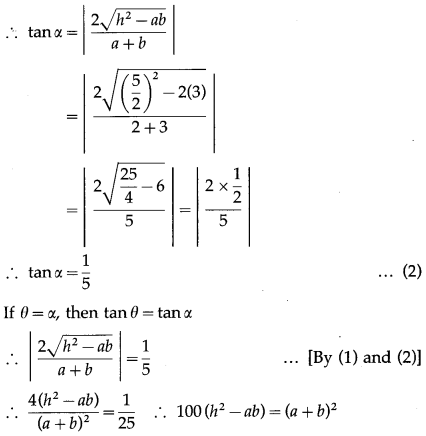This is the required condition.Question 7.
Find the combined equation of lines passing through the origin and each of which making angle 60° with the Y- axis.
Solution: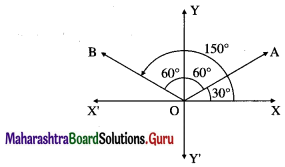Let OA and OB be the lines through the origin making an angle of 60° with the Y-axis.
Then OA and OB make an angle of 30° and 150° with the positive direction of X-axis.
∴ slope of OA = tan 30° = $$\frac{1}{\sqrt{3}}$$
∴ equation of the line OA is
y = $$\frac{1}{\sqrt{3}}$$ = x, i.e. x – $$\sqrt {3}$$y = 0
Slope of OB = tan 150° = tan (180° – 30°)
= tan 30° = $$-\frac{1}{\sqrt{3}}$$
∴ equation of the line OB is
y = $$-\frac{1}{\sqrt{3}}$$x, i.e. x + $$\sqrt {3}$$ y = 0
∴ required combined equation is
(x – $$\sqrt {3}$$y)(x + $$\sqrt {3}$$y) = 0
i.e. x2 – 3y2 = 0.